# List Sheet Names with Formula – Excel & Google Sheets

This tutorial demonstrates how to list the sheet names of a workbook with a formula in Excel.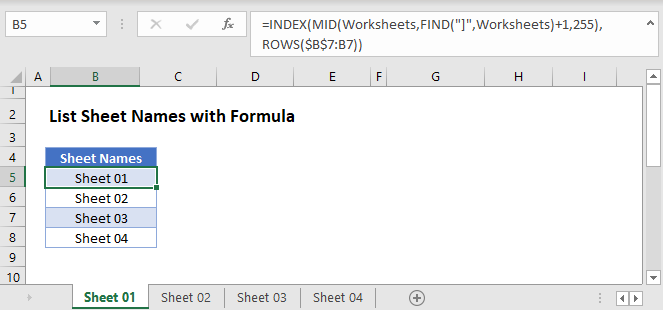## List Sheet Names Using Named Range and Formula

There is no built-in function in Excel that can list all the worksheets in a workbook. Instead you have two options:

1. Use a VBA Macro to list all sheets in the workbook
2. Create a Formula to list all sheets

If you want to use a formula, follow these steps:

1. Create a named range “Worksheets”
2. Use a formula to list out all sheet names.

### Create Name Range for Sheet Names

To create a Named Range for the sheet names, in the Excel Ribbon: Formulas > Name Manager > New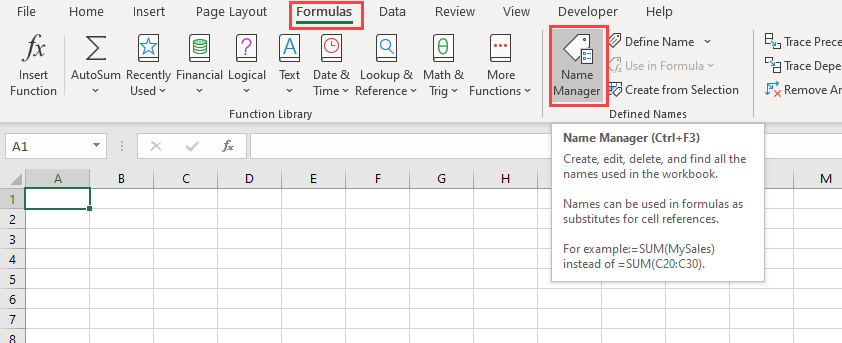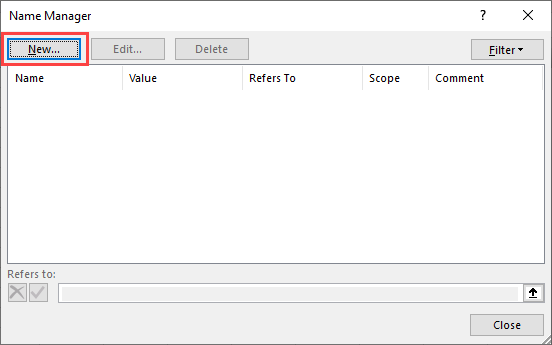Type “Worksheets” in the Name Box: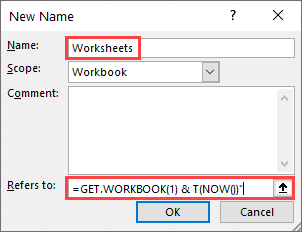In the “Refers to” section of the dialog box, we will need to write the formula

``=GET.WORKBOOK(1) & T(NOW())"``

This formula stores the names of all sheets (as an array in this format: “[workbook.xlsm].Overview”) in the workbook to the named range “Worksheets”.

The “GET.WORKBOOK” Function is a macro function, so your workbook has to be saved as a macro-enabled workbook (file format: .xlsm) for the sheet names to be updated each time the workbook is opened.

Note: When filling the Edit name dialog box, workbook should be selected as the scope of the name range.

### Using Formula to List Sheet Names

Now we use a formula to list the sheet names. We’ll need the INDEX, MID, FIND, and ROWS Functions:

``=INDEX(MID(Worksheets,FIND("]",Worksheets)+1,255),ROWS(\$B\$5:B5))``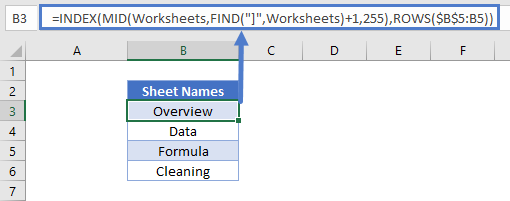• The formula above takes the “Worksheets” array and displays each sheet name based on its position.
• The MID and FIND Functions extract the sheet names from the array (removing the workbook name).
• Then the INDEX and ROW Functions display each value in that array.
• Here, “Overview” is the first sheet in the workbooks and “Cleaning” is the last.

## Alternate Method

You also have the option to create the list of sheet names within the Name Manager. Instead of

``=GET.WORKBOOK(1) & T(NOW())``

set your “Refers to” field to

``=REPLACE(GET.WORKBOOK(1),1,FIND("]",GET.WORKBOOK(1)),"")``

Now there’s no need for MID, FIND, and ROWS in your formula. Your named range is already made up of only sheet names.

Use this simpler INDEX formula to list the sheets:

``=INDEX(SheetName,B3)``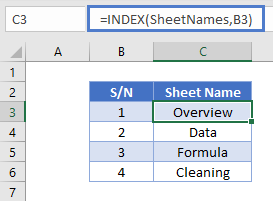### Excel Practice Worksheet

Practice Excel functions and formulas with our 100% free practice worksheets!